# Learning to Be Less Helpful

FREDERICK PECK

University of Montana, Missoula

Dan Meyer is as close as we can get to a rock star in the world of mathematics education. These days, Dan is known for many things: 3-act tasks, 101 Q’s, Desmos, NCTM’s ShadowCon, to name just a few. But he initially rose to prominence on the basis of a TEDTalk that he gave as a high school teacher in 2010 (I recommend it highly). In his talk, he speaks directly to math teachers, and gives one simple piece of advice.

Teachers often understand their job as involving “help,” in some form or another. Most of us would like to think that we help students learn. Dan’s advice can therefore be understood as a challenge to a core aspect of our identity. And yet, the advice has become a mantra of sorts for teachers at all levels.

What does it mean to be less helpful, and why should that be a goal?

In his TED Talk, Dan shows how interesting mathematical questions are often surrounded by scaffolding: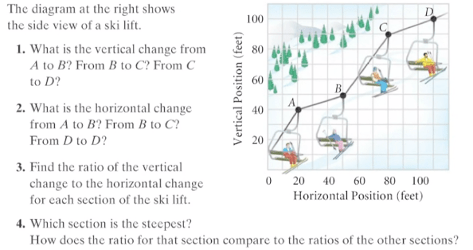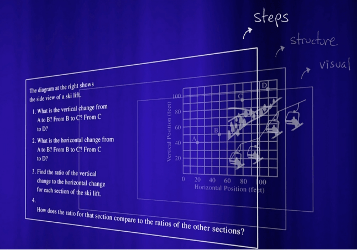This scaffolding, in the form of mathematical structure and sequences of steps, works to obscure the interesting question (“which section is the steepest?”, buried in question 4). Students are asked to apply a mathematical structure (a coordinate plane) and accomplish sub goals (e.g., find vertical and horizontal distances) before they even know the goal (find the steepest section). Collectively, the scaffolding works to make math seem like an exercise in rule-following, rather than an opportunity to explore and make sense of interesting questions.

More perniciously, the scaffolding takes away much of the mathematics. Hans Freudenthal believed that structuring was at the heart of mathematics. In this problem, the scaffolding has already structured the problem. There is no structuring—and therefore no mathematics—left for the student to do. By presenting students with a ready-made structure for getting an answer, “mathematics” is reduced to answer-getting.  To be less helpful means to remove the scaffolding, and to let students do mathematics.  That is hard work for a teacher. In this post, I’ll give an example from my own practice, and discuss how I’m learning to be less helpful.

# Background

I have always considered myself a “reform-oriented” teacher—at least in content courses, my classroom practice adheres closely to the “conceptual math” described by Amanda Serenevy in an earlier post on this blog. In calculus, I have followed many of the recommendations from the calculus reform movement of the 1990s, including the “rule of four”: concepts and procedures should be explored from graphical, numerical, algebraic, and verbal perspectives. For example, early in the year, before any formal procedures are introduced, students explore derivatives graphically and verbally:Following the rule of four (actually, I also use a lot of models, so let’s say, the rule of five) we use many strategies to develop differentiation rules and make connections between them. We use numerical analysis (looking at successive differences) to make conjectures about derivatives of polynomials. We couple this with verbal and graphical analyses (such as the examples above), which we also used to make conjectures about the derivatives of sin(x) and cos(x). We use first principles (algebraic) to prove these conjectures about elementary functions. We use models (area and see-saw, respectively) to develop the product rule and chain rule. We use algebraic strategies to write unknown situations in terms of known situations. This allows us to develop new rules, e.g., the quotient rule by rewriting a quotient as a product: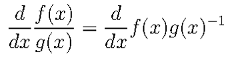and to find derivatives of new functions (e.g., developing the derivative of tan x by writing it as sin x / cos x . Later in the course we develop the logarithm as an accumulator function, and thus we can use the fundamental theorem to find the derivative,.

Finally, we come to a point in the course where it’s time to find the derivative of exponential functions. This is one of the crown jewels in a first-semester calculus course and I want to ensure that students understand it conceptually. As a first-year teacher, my plan was as follows:

1. Using the rule of four, students would explore the derivative of y=2x from graphical, numeric, algebraic, and verbal perspectives.
1. Putting these perspectives together, students would conjecture that the derivative of  y=2x is itself an exponential function—in fact, it is a vertical compression of the original function, with a scale factor of about 0.69. That is:
1. Do a similar analysis for  y=5x. Here we come to a similar conjecture, only now the vertical scaling is a stretch, rather than a compression:
1. Putting these two conjectures together leads to three interesting observations.
Observation: The derivative of an exponential function seems to be a vertically-scaled exponential function with the same base.Observation: Some scale factors are <1, and they compress the derivative, relative to the initial function (e.g., the scale factor for y=2x). Other scale factors are >1, and they stretch the initial function (e.g., the scale factor for y=2x).Observation: Bigger bases seem to have bigger scale factors.
2. These observations, in turn, lead to a very interesting question. If the derivative of 2x  is a compression of the initial function (a<1), and the derivative of 5x is a stretch of the initial function (a>1), and if the scale factor generally grows as the base grows, then reasoning by continuity tells us that there must be a base between 2 and 5 where the scale factor is exactly 1.  What is this magical base?
3. Using technology, explore this question. Can we find such a base?

This plan hinges on students developing a good sense of the behavior of the derivative of 2x, that is, steps 1 and 2. Thus, for the rest of this post, I’ll limit my discussion to these steps.

# My first attempt

In my first attempt at steps 1 and 2, students competed this worksheet in groups.  The worksheet guides students to consider the derivative of 2x from a variety of perspectives.

## Graphical:## Numerical:## Algebraic: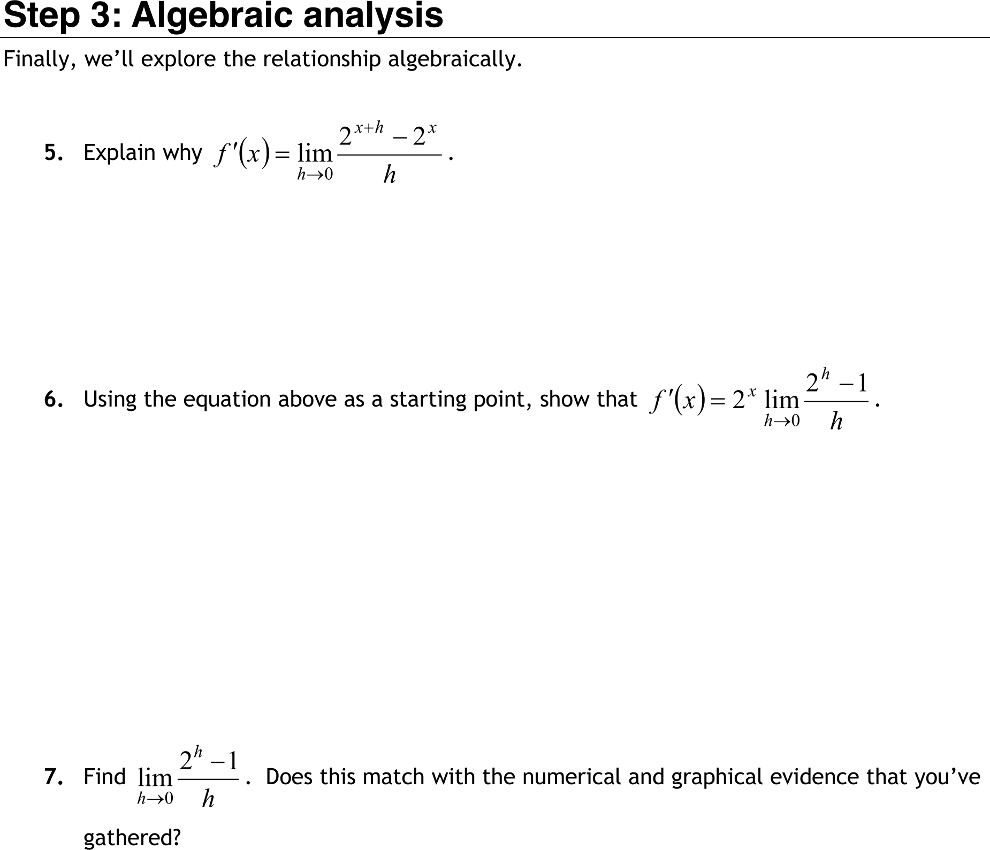## Verbal:

Throughout, students are asked to reflect on or explain their findings.  The worksheet culminates with a prompt to make a conjecture about the derivative of 2x: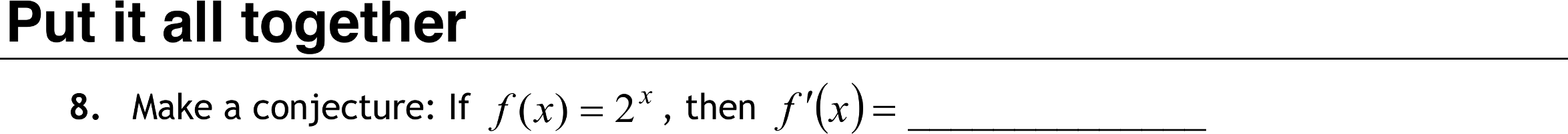Can you guess what happened at this point?

Well, let me tell you what didn’t happen. Students didn’t take a holistic perspective on the work that they had done. They didn’t say things like, “from step 1, I see that the graph of the derivative is a vertical compression of 2x. From step 2, I see that the ratio of the derivative to the initial function is about 0.69. From step 3, I see that f ′(x) is some constant times 2x, and I see that the constant is 0.69. Taken together, I conjecture that the.”

Instead, students treated question 8 as if it were independent from the rest of the worksheet. They generally conjectured that the derivative of 2x was x⋅2x-1 (i.e., they used the power rule). Even though students had just completed a graphical, numeric, analytic, and verbal analysis of the derivative of 2x, they did not draw on this analysis in answering the big question.

I tried this worksheet for a few years, each time with the same result. What I finally understood was that I needed to be less helpful. Even though I saw the mathematical structure in the worksheet, students didn’t. This is because I was the one who was doing the structuring. I created a cookbook-style “recipe for mathematics,” and in doing so, I had done all of the mathematics. The only thing that was left for students to do was to follow steps and get answers.

# Deciding to be less helpful

After a few of years of this worksheet, always with the same good intentions, always with the same disappointing results, I finally took Dan’s advice to heart. I decided to be less helpful.

Dan suggests that we start with the big question rather than burying it under a pile of scaffolding. My big question was, what is the derivative of 2x?

So that’s what I did. I put the question on the board, and asked students to explore it. I was really nervous, because I had never been this unstructured before. Structure is comforting to a teacher. Removing it is risky.

Now, can you guess what happened?

Actually, the same thing as when students got to question 8 on the worksheet. They applied the power rule and thought they were finished. Even though we had previously used graphical, numeric, and analytic approaches for finding derivatives of unknown functions, students did not use them here. In fact, they did not even recognize that I had asked a “big question.” Rather, my question seemed to them to be a straightforward application of a known rule.

I learned that I needed to set up the question better.

In math education, we call the setup to a problem the “launch.” Recently researchers have identified the launch as a key phase in a successful problem-solving lesson. When done well, the launch prepares students to engage in a complex problem. When done poorly, the launch either gives away too much, decreasing the cognitive demand of the problem, or doesn’t provide enough grounding, limiting students’ ability to engage in the problem.

A good launch should do three things:

1. Help make sure that all students are familiar with the context and content of the problem
2. Produce a perplexing question
3. Remind students about relevant prior knowledge without “giving away” the solution or solution strategy

Here is how my launch works now:

1. Just ask it. Ask students to find the derivative of y=2x.
2. When the majority of the class produces x⋅2x-1 as the derivative, have them check this by graphing their conjecture against the numerical derivative produced by their calculators.
3. When the graphs do not match, this produces a perplexing question: What’s going on here, and what is the actual derivative of y=2x ?
4. Before asking students to explore this question, I give a brief presentation that summarizes our previous strategies for finding derivatives of unknown functions: numerical analysis, graphical analysis, algebraic analysis using first principles, and algebraic analysis by rewriting the unknown function as a combination of known functions.
5. Just ask it, redux. What is the derivative of 2x?

In my experience, this sequence accomplishes the objectives of a good launch. Students now see the question about the derivative of 2x as a “big question,” worthy of exploration, and they have tools that they can use to explore that question. At the same time, I haven’t told them what to do or what the answer is, and so there is still plenty of mathematics to be done by students. The evidence of a successful launch is deep student engagement in the problem, and that has been my experience with this launch.

Groups go at the problem in different ways.

I don’t get every approach every year, but each year I get at least a few of these approaches. I have students share their approaches, and we make connections between them as a class. By the end of our class discussion, students are ready to conjecture that,  and I can continue with the lesson. All together, this part of the lesson takes about 50 minutes: 10 minutes for the launch, 20 minutes for group exploration, 10 minutes for group presentations, and 10 minutes for me to summarize and connect approaches.

# Learning to be less helpful

I’m learning that when I’m less helpful, students learn more. This is because rather than going through steps that are initially disconnected from a larger goal, students are consciously engaged in exploring a big question right from the beginning.

I’m learning that a lot of mathematics materials are cookbook-style “recipes for mathematics,” much like my initial worksheet was1. I’m learning to use these materials to identify the key question, and then be less helpful by removing much of the scaffolding.

I’m learning that being less helpful is risky. Being less helpful means giving some of the control of the class over to students. Not all students will use every approach in their groups (unlike in my worksheet, where every student did the same thing). Instead, groups have discretion about the strategies that they use. And because the class discussion is dependent on the group work that came before it, the particular strategies that one class sees may differ from those that another class sees. There is less structure and more heterogeneity.

I’m learning that heterogeneity can be a resource. I depend on different groups taking different approaches. This allows me to structure our follow-up discussion so that students can see the connections between graphical, numeric, and algebraic approaches.

I’m learning that it actually takes a lot of work to be less helpful:

• Sometimes it takes work to develop the right question—a question that is perplexing, that is challenging yet solvable, and that is likely to lead students to develop the targeted mathematics. In this example, the question was somewhat obvious, but it is not always so easy.
• It takes a lot of work to develop the right launch.
• It takes work to sequence student strategies into a coherent narrative, including analyzing unforeseen strategies on the fly and incorporating them into the emerging narrative.

I’m learning that being less helpful doesn’t mean that I can’t help students. During group work, I often prompt students to develop their strategy further or connect it to a different strategy. Sometimes I’m pretty explicit in this prompting. For example, I’ll suggest that students try to make a table. Sometimes, if no group produces a particular strategy, I’ll introduce it in the class discussion.

I’m learning that being less helpful isn’t a magic bullet—not all of my students are engaged all of the time or learn all I’d want them to learn. But when I have a good question and a good launch, more students are more engaged in the activity and learn more of the mathematics than when I do the structuring for them.

I’m learning that one can learn to be less helpful. These days, it doesn’t feel so risky to ask the big question about 2x, because I have a very good sense of what will happen. And it doesn’t feel quite so risky to ask new and different big questions. I know that I’ll fail sometimes (as in when I just ask the big question with no launch), but I also know that this is part of the process.

1 This includes many so-called “reform-oriented” materials. It’s likely that many reformers share my goal of engaging students in activity, and are trying desperately to shed the urge to explain—the traditional lay-it-all-out-and-then-let-the-student-practice approach, in which none of the puzzling-through is left for the student. However, it seems that often reform-oriented materials replace traditional explanations with a series of leading questions. Much like in my initial approach, these questions do all of the structuring for students and leave little mathematics for students to do.

This entry was posted in Active Learning in Mathematics Series 2015, Classroom Practices. Bookmark the permalink.

### 1 Response to Learning to Be Less Helpful

1.Victoria says:

Thank you so much for sharing this! I am a student teacher this year and I’m struggling to find the balance of scaffolding instructions vs. letting students figure it out on their own. This was extremely helpful and I can’t wait to implement this in my classroom.